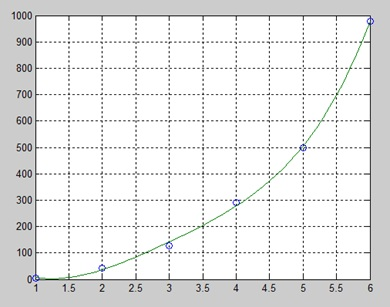# MATLAB - Polynomials

#### Data Preprocessing for Machine Learning using MATLAB

30 Lectures 4 hours

#### Image Processing Toolbox in MATLAB

17 Lectures 3 hours

#### Matlab - The Complete Course

Best Seller

37 Lectures 5 hours

MATLAB represents polynomials as row vectors containing coefficients ordered by descending powers. For example, the equation P(x) = x4 + 7x3 - 5x + 9 could be represented as −

p = [1 7 0 -5 9];

## Evaluating Polynomials

The polyval function is used for evaluating a polynomial at a specified value. For example, to evaluate our previous polynomial p, at x = 4, type −

```p = [1 7 0  -5 9];
polyval(p,4)
```

MATLAB executes the above statements and returns the following result −

```ans = 693
```

MATLAB also provides the polyvalm function for evaluating a matrix polynomial. A matrix polynomial is a polynomial with matrices as variables.

For example, let us create a square matrix X and evaluate the polynomial p, at X −

```p = [1 7 0  -5 9];
X = [1 2 -3 4; 2 -5 6 3; 3 1 0 2; 5 -7 3 8];
polyvalm(p, X)
```

MATLAB executes the above statements and returns the following result −

```ans =
2307       -1769        -939        4499
2314       -2376        -249        4695
2256       -1892        -549        4310
4570       -4532       -1062        9269
```

## Finding the Roots of Polynomials

The roots function calculates the roots of a polynomial. For example, to calculate the roots of our polynomial p, type −

```p = [1 7 0  -5 9];
r = roots(p)
```

MATLAB executes the above statements and returns the following result −

```r =
-6.8661 + 0.0000i
-1.4247 + 0.0000i
0.6454 + 0.7095i
0.6454 - 0.7095i
```

The function poly is an inverse of the roots function and returns to the polynomial coefficients. For example −

```p2 = poly(r)
```

MATLAB executes the above statements and returns the following result −

```p2 =

Columns 1 through 3:

1.00000 + 0.00000i   7.00000 + 0.00000i   0.00000 + 0.00000i

Columns 4 and 5:

-5.00000 - 0.00000i   9.00000 + 0.00000i
```

## Polynomial Curve Fitting

The polyfit function finds the coefficients of a polynomial that fits a set of data in a least-squares sense. If x and y are two vectors containing the x and y data to be fitted to a n-degree polynomial, then we get the polynomial fitting the data by writing −

```p = polyfit(x,y,n)
```

### Example

Create a script file and type the following code −

```x = [1 2 3 4 5 6]; y = [5.5 43.1 128 290.7 498.4 978.67];   %data
p = polyfit(x,y,4)   %get the polynomial

% Compute the values of the polyfit estimate over a finer range,
% and plot the estimate over the real data values for comparison:
x2 = 1:.1:6;
y2 = polyval(p,x2);
plot(x,y,'o',x2,y2)
grid on
```

When you run the file, MATLAB displays the following result −

```p =
4.1056  -47.9607  222.2598 -362.7453  191.1250
```

And plots the following graph −